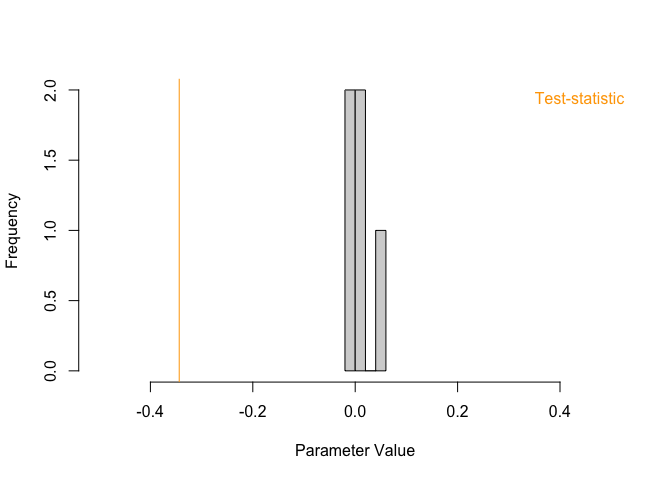# mlVAR Permutation Test

The mnet package can be installed from Github using the devtools package:

# library(devtools)
# install_github("jmbh/mnet")
library(mnet)

To illustrate the permutation test for group differences in multilevel VAR models we use simulated data that is included in the mnet package. The data consists of two groups with 100 subjects each, with time series of three variables of length 100 for each subject. The data were generated from a mlVAR model in which one of the cross-lagged effects (2->1) is different across groups.

data("ExampleData")

dim(ExampleData)
>  20000     5
>           V1         V2         V3 id group
> 1 -0.5503574  2.3730731 -0.9546494  1     1
> 2 -0.8676930  2.6043886  0.1419776  1     1
> 3 -1.0098863  1.9953243  0.8989440  1     1
> 4 -2.2597404  1.5433871  0.2017678  1     1
> 5 -1.4791477 -0.3571881 -0.6923630  1     1
> 6 -0.6629657 -0.2803782 -0.1600937  1     1

The mlVAR_CG() function implements the permutation test and has the same input arguments as the mlVAR() function from the mlVAR package, except that it requires two data arguments for the two groups and requires specifying the number of permutations the test should be based on. The more permutations, the more precisely p-values can be computed.

We provide the two datasets to mlVAR_CG(), and as in mlVAR(), we specify the column names of the variables that should be modeled with the argument vars and indicate the column with the unique identifiers for each subject with the argument idvar. Finally, we indicate the column indicating the group membership with the argument group,

set.seed(1) # reproducibility

timer <- proc.time()

output <- mlVAR_GC(data = ExampleData,
vars = c("V1", "V2", "V3"),
idvar = "id",
groups = "group",
nCores = 1, # choose cores available on your machine
nP = 5, # Should be much more in practice, see preprint!
pbar = FALSE)
> Warning: executing %dopar% sequentially: no parallel backend registered

proc.time() - timer
> elapsed
>  72.435

The perhaps most relevant output are the observed group differences and their associated p-values. In the current version, we consider group differences in between-person networks, in VAR networks (both fixed effects and random effects standard deviation) and the contemporaneous networks (both fixed effects and random effects standard deviation). The observed group differences in all these networks can be found in out$EmpDiffs. All group differences are Group1 - Group2. output$EmpDiffs
> $Lagged_fixed > , , 1 > > V1 V2 V3 > V1 -0.00252620 -0.343710677 0.019928177 > V2 0.02702579 0.009729344 -0.002083037 > V3 -0.01797542 -0.010346780 -0.002122644 > > >$Lagged_random
> , , 1
>
>               V1           V2         V3
> V1 -0.0001694804  0.033679705 -0.0133229
> V2 -0.0247214500 -0.017000309  0.0253878
> V3 -0.0199360377 -0.009975728  0.0285887
>
>
> $Contemp_fixed > V1 V2 V3 > V1 0.000000000 -0.005761086 -0.01054800 > V2 -0.005761086 0.000000000 0.01351376 > V3 -0.010548003 0.013513757 0.00000000 > >$Contemp_random
>             V1          V2          V3
> V1  0.00000000 -0.00246086  0.01120576
> V2 -0.00246086  0.00000000 -0.04474056
> V3  0.01120576 -0.04474056  0.00000000
>
> $Between > V1 V2 V3 > V1 0.0000000 -0.65312918 0.15266785 > V2 -0.6531292 0.00000000 0.06451328 > V3 0.1526679 0.06451328 0.00000000 The associated p-values can be found in output$Pval
> $Lagged_fixed > [,1] [,2] [,3] > [1,] 0.8 0.0 0.4 > [2,] 0.0 0.8 1.0 > [3,] 0.8 0.6 1.0 > >$Lagged_random
>      [,1] [,2] [,3]
> [1,]  1.0  0.0  0.6
> [2,]  0.4  0.8  0.0
> [3,]  0.4  0.6  0.2
>
> $Contemp_fixed > [,1] [,2] [,3] > [1,] NA NA NA > [2,] 0.6 NA NA > [3,] 0.8 0.2 NA > >$Contemp_random
>      [,1] [,2] [,3]
> [1,]   NA   NA   NA
> [2,]    1   NA   NA
> [3,]    1  0.8   NA
>
> $Between > [,1] [,2] [,3] > [1,] NA NA NA > [2,] 0.0 NA NA > [3,] 0.2 0.8 NA For example, we see that the group difference for the cross lagged effect 2->1 is significant. Note that the p-value is here exactly zero, because we computed p-values based on empirical sampling distribution. If the test-statistic is far away from the mass of the sampling distribution under the null hypothesis (no group difference), then the density of the sampling distribution in this area is extremely low and therefore unlikely to be sampled. This leads to p-values that are exactly zero. We can also inspect the exact sampling distributions. Let’s again consider the cross lagged effect 2->1: hist(output$SampDist$Lagged_fixed[1,2,], xlim=c(-.5, .5), main="", xlab="Parameter Value") abline(v=output$EmpDiffs\$Lagged_fixed[1,2,], col="orange")
legend("topright", legend="Test-statistic", text.col="orange", bty="n")We see that the test-statistic is not even overlapping with the support sampling distribution, which gives a p-value of zero as discussed above.